﻿ 基于STAR-CCM+的某SUV汽车热舒适的研究

# 基于STAR-CCM+的某SUV汽车热舒适的研究Research on Thermal Comfort of an SUV Based on STAR-CCM+

Abstract: Taking an SUV 6-seat pure electric vehicle as the research object, the cold and comfort of the whole vehicle are studied by simulation. The human body model is introduced, and the thermal environ-ment comfort in the passenger compartment is analyzed and evaluated by using PMV-PPD and MAA. The simulation results show that the distribution of cold air velocity and passenger body surface temperature in the cabin is comfortable. Through the analysis of the distribution of evaluation in-dicators, we can clearly understand the distribution of comfort in the passenger compartment, which has the advantages of more cost saving and shorter time effectiveness than the experiment.

1. 引言

2. 模型的建立

2.1. 几何模型及网格划分

2.2. 数学模型

STAR-CCM+中有自带的数值求解器，可以精确模拟车内的热舒适情况。车内空气流速较小，使用k-ε湍流模型，内部流体可看作三维不可压缩流 ；乘客舱内的空气为辐射透明介质，符合灰体辐射。求解方程如下：

$\frac{\partial \rho }{\partial t}+\frac{\partial \rho {u}_{i}}{\partial {x}_{i}}=0$ (1)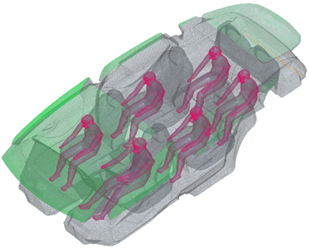Figure 1. Curve: system result of standard experiment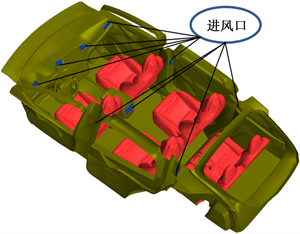Figure 2. Curve: system result of standard experiment

$\frac{\partial \rho {u}_{i}}{\partial t}+\frac{\partial \rho {u}_{i}{u}_{j}}{\partial {x}_{i}}=-\frac{\partial P}{\partial {x}_{i}}+\frac{\partial }{\partial {x}_{j}}\left(\mu \left(\frac{\partial u}{\partial {x}_{j}}+\frac{\partial {u}_{j}}{\partial {x}_{i}}\right)\right)$ (2)

$\frac{\partial \rho T}{\partial t}+\frac{\partial \rho T{u}_{j}}{\partial {x}_{j}}=\frac{\partial }{{c}_{p}\partial {x}_{i}}\left(k\frac{\partial T}{\partial {X}_{i}}\right)$ (3)

3. 边界参数及评价指标

3.1. 边界参数

3.2. 评价指标

3.2.1. PMV-PPD

PMV表示预期平均热感觉，其将舒适状态从冷到热划分为7个等级，见表1。由于环境的舒适性因人体的生理情况不同而异。所以不适人员比例PPD被提出来表达人们对热环境的不满意程度，当PMV在−0.5~0.5时，且PPD < 15%时，人体处于舒适状态。PMV和PPD的计算如式(4)和(5)。

$\text{PMV}=\left[0.028+0.303\mathrm{exp}\left(-0.036M\right)\right]\cdot \left[M-W-{E}_{c}-{E}_{res}-{C}_{res}-H\right]$ (4)

$\text{PPD}=100-95\cdot \mathrm{exp}\left(-0.03353\cdot {\text{PMV}}^{4}-0.2179\cdot {\text{PMV}}^{2}\right)$ (5)

W——人做的机械功，W/m2

${E}_{c}$ ——表皮及出汗的散热量，W/m2

${E}_{res}$ ——呼吸潜热损失量，W/m2

${C}_{res}$ ——呼吸显热损失量，W/m2

H——体表对流和辐射热损失，W/m2Table 1. Comfort level of PMV evaluation index

3.2.2. 空气龄(MAA)

$\tau =\frac{{\int }_{0}^{\infty }C\left(\tau \right)\text{d}\tau }{{C}_{0}}$ (6)

$C\left(\tau \right)$ ——当以某种特定送风方式送入新鲜空气时测得的示踪气体浓度随时间的变化。

4. 模拟结果分析

4.1. 乘客舱空气流速分布情况

4.2. 乘客舱成员体表温度分布情况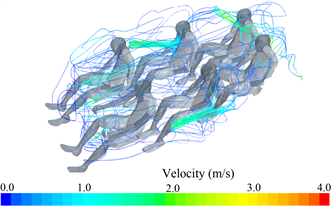Figure 3. Schematic diagram of air flow line in vehicle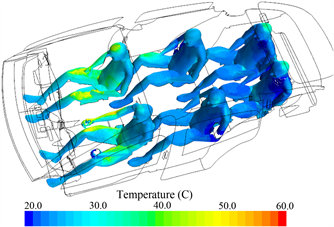Figure 4. Passenger surface temperature

4.3. 乘客舱平均温度分布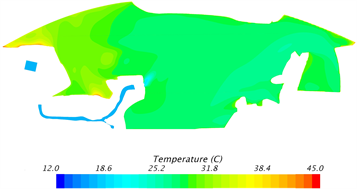Figure 5. Cross section temperature inside vehicle

4.4. PMV-PPD评价指标分析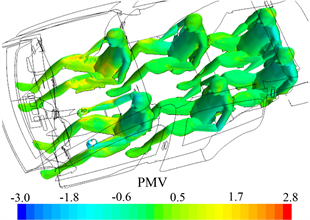Figure 6. PMV distribution on passenger surface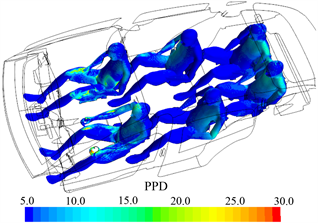Figure 7. PPD distribution on passenger surface

4.5. 空气龄(MAA)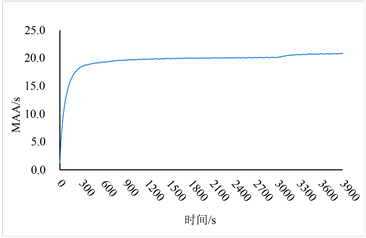Figure 8. RH distribution of passenger surface relative humidity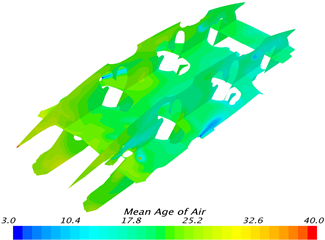Figure 9. RH distribution of passenger surface relative humidity

5. 总语

 Mezrhab, A. and Bouzidic, M. (2005) Computation of Thermal Comfort inside a Passenger Car Compartment. Applied Thermal Engineering, 26, 1697-1704.
https://doi.org/10.1016/j.applthermaleng.2005.11.008

 林飞跃. 城市客车热舒适性空调系统结构改进[J]. 客车技术与研究, 2019, 41(3): 25-27.

 杨志刚, 徐鑫, 赵兰萍, 林赵敏. 冬季夜间乘员舱内热环境及人体热舒适性研究[J]. 同济大学学报(自然科学版), 2019, 47(3): 408-413.

 杨金凤, 符永正, 周传辉. 一个中型会议室空气龄和舒适度的数值模拟研究[C]//中国制冷学会, 湖北省制冷学会, 湖北省土木建筑学会. 2007年湖北省暖通空调制冷及热能动力学术年会论文集, 2008: 159-163.

 宋晓峰, 黄学章. 风道出风口结构对客车车内流场影响的研究[J]. 客车技术与研究, 2018, 40(5): 8-11.

 Noor, E.A.S., Haslinda, M.K. and Nazri, K. (2015) CFD Simulation of Air Temperature Inside a Bus Passenger Compartment. Applied Mechan-ics and Materials, 735, 85-90.
https://doi.org/10.4028/www.scientific.net/AMM.735.85

 周柯. 纯电动公交车内气流分布特性及热舒适性研究[D]: [硕士学位论文]. 成都: 西南交通大学, 2017.

 杨春丽, 刘艳. 单开口有限空间自然通风特性及其影响因素研究[J]. 安全与环境工程, 2019, 26(4): 183-189.

 张炳力, 薛铁龙, 胡忠文. 基于PMV-PPD与空气龄的轿车乘员舱内热舒适性分析与改进[J]. 汽车工程, 2015, 37(8): 951-958.

 谢普康, 陈洪涛, 商潭苏, 邢首辰, 刘方圆, 林好利. 基于STAR-CCM+的某型拖拉机驾驶室热舒适性研究[J]. 拖拉机与农用运输车, 2016, 43(3): 14-17.

 David, M., Francisco, J., Carlos, B. and Guerra, J.J. (2014) The De-velopment and Validation of a Thermal Model for the Cabin of a Vehicle. Applied Thermal Engineering, 66, 646-656.
https://doi.org/10.1016/j.applthermaleng.2014.02.054

 Christian, D., Walter, S. and Martin, K. (2015) A Mod-ular Thermal Simulation Tool for Computing Energy Consumption of HVAC Units in Rail Vehicles. Applied Thermal Engineering, 78, 616-629.
https://doi.org/10.1016/j.applthermaleng.2014.11.065

 张竹慧. 室内平均辐射温度的建筑设计与调节[J]. 山西建筑, 2009, 35(16): 183-184.

 Barbara, T.-J., Filip, B., Jose, M., Di Sciullo, F. and Payá, J. (2015) Transient Thermal Model of a Vehicle’s Cabin Validated under Variable Ambient Conditions. Applied Thermal Engineering, 75, 45-53.
https://doi.org/10.1016/j.applthermaleng.2014.05.074

 Liu, T., Liu, Z., Li, G. and Zuo, Z. (2015) Comparative Study of Numerical Simulation of Indoor Thermal Environment in the Pattern of Personalized Ventilation and Stratum Ventilation. Procedia Engineering, 121, 785-791.
https://doi.org/10.1016/j.proeng.2015.09.031

Top